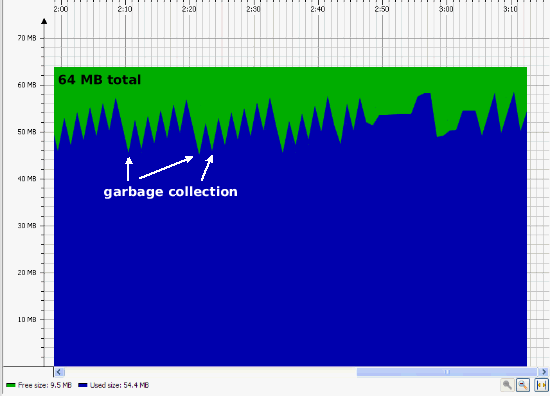# Calling Java from MATLAB, Memory Issues

Following up with my previous post about using Java objects in MATLAB, this week I’m going to discuss the memory issues that can crop up.

When a Java object is created in the MATLAB workspace, it is actually created in the Java heap space and not the main memory used by MATLAB. On a 32-bit windows machine, MATLAB might have up to 3 GB available for creating matrices and other MATLAB objects but only 64 or 128 MB (by default) for creating java objects. To find out how much memory is available for creating Java objects, there are three numbers of importance: the free memory, the total memory, and the max memory.

The free memory is the number of bytes available in the heap space for creating new objects. The total memory is the number of bytes allocated to the total heap space which is the size of the objects created + the free memory. When the amount of free memory starts to get low, java will increase the total memory until it equals the the max memory. You can see this in the following animated graph, where the green (total memory) is bumped up when I create the large array of java doubles. The jumps in the blue represent the objects created as I manipulated the editor and command window to set up this demo, and the dips are when those objects get garbage collected, freeing up memory. The actual double array isn’t shown on this graph as it is created and cleared faster than the sampling time of the graph.This is the demo that was running at the time of the jump: you can see the commands to discover the java memory, as well as the fact that the array creation ups the used java memory but does not affect the memory for MATLAB array creation:

free = java.lang.Runtime.getRuntime.freeMemory
total = java.lang.Runtime.getRuntime.totalMemory
max = java.lang.Runtime.getRuntime.maxMemory
feature('memstats')
x = javaArray('java.lang.Double',400000);
for i=1:400000
x(i)=java.lang.Double(i);
end
free =

13271784

total =

65470464

max =

130875392

Physical Memory (RAM):
In Use:                             1911 MB (77755000)
Free:                               1655 MB (677f0000)
Total:                              3567 MB (def45000)
Page File (Swap space):
In Use:                             2205 MB (89dd3000)
Free:                               3243 MB (cab14000)
Total:                              5448 MB (1548e7000)
In Use:                              585 MB (24928000)
Free:                               1462 MB (5b6b8000)
Total:                              2047 MB (7ffe0000)
Largest Contiguous Free Blocks:
1. [at 320b5000]                    459 MB (1cb9b000)
2. [at 4edf6000]                    279 MB (117da000)
3. [at 21810000]                    263 MB (107f0000)
4. [at 605d9000]                    146 MB ( 9217000)
5. [at 6d346000]                     71 MB ( 470a000)
6. [at 697f6000]                     58 MB ( 3a3a000)
7. [at 71ce7000]                     19 MB ( 1319000)
8. [at 73026000]                     18 MB ( 126a000)
9. [at 7e4a1000]                     18 MB ( 124f000)
10. [at 7d1d7000]                     18 MB ( 1239000)
======= ==========
1352 MB (548cb000)

ans =

481931264


free = java.lang.Runtime.getRuntime.freeMemory
total = java.lang.Runtime.getRuntime.totalMemory
max = java.lang.Runtime.getRuntime.maxMemory
feature('memstats')
free =

31137576

total =

102739968

max =

130875392

Physical Memory (RAM):
In Use:                             1919 MB (77f2a000)
Free:                               1648 MB (6701b000)
Total:                              3567 MB (def45000)
Page File (Swap space):
In Use:                             2238 MB (8be27000)
Free:                               3210 MB (c8ac0000)
Total:                              5448 MB (1548e7000)
In Use:                              584 MB (248b8000)
Free:                               1463 MB (5b728000)
Total:                              2047 MB (7ffe0000)
Largest Contiguous Free Blocks:
1. [at 320b5000]                    459 MB (1cb9b000)
2. [at 4edf6000]                    279 MB (117da000)
3. [at 21810000]                    263 MB (107f0000)
4. [at 605d9000]                    146 MB ( 9217000)
5. [at 6d346000]                     71 MB ( 470a000)
6. [at 697f6000]                     58 MB ( 3a3a000)
7. [at 71ce7000]                     19 MB ( 1319000)
8. [at 73026000]                     18 MB ( 126a000)
9. [at 7e4a1000]                     18 MB ( 124f000)
10. [at 7d1d7000]                     18 MB ( 1239000)
======= ==========
1352 MB (548cb000)

ans =

481931264



One caution is that this space is shared between any objects you might create as well as objects created by the Desktop, Editor, Current Folder, etc. Once all the java memory is used up, there is the potential of locking up MATLAB, as there might not be enough room to even create an out-of-memory dialog.

There is a solution to running out of Java memory that is usually safe, although sometimes produces unintended consequences if the numbers are made too large. That is to create or modify a java.opts file in the MATLAB startup directory. This allows you to pass JVM options (such as the total and max memory for the heap) on MATLAB startup. Instructions can be found at this solution.

|